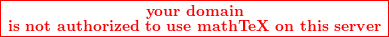# Frobenius series

Why do we assume that the first term c0 in a frobenius seriescannot equal 0?

Thanks!

Ben Niehoff
Gold Member
Latex seems to be misbehaving, so I'll write in plain text:

In the Frobenius substitution, the x dependence of the first term is already factored out:

y(x) = x^r Sum (a_k x^k)

So, the first term in the series is actually

a_0 x^r

and when we plug the series into the differential equation, the question we are asking is "What is the smallest r for which a_0 does not vanish?" The answer is given by the indicial equation.

After solving the indicial equation for r, we are then equipped to ask the next question: "Given that a_0 does not vanish, can I find some sequence a_k such that my formal sum converges and solves the differential equation?"

Thanks for the response. Why is it necessary though that a0 not vanish?

Thanks for the response. Why is it necessary though that a0 not vanish?

Let say the first term that we obtained on substituting the Frobenius series into the DE as

Aa0xr + .... is identically zero.

This implies Aa0=0.
We may assume a0 to be zero or nonzero. But if it is zero then A can be any number. Not an interesting result.

Last edited: